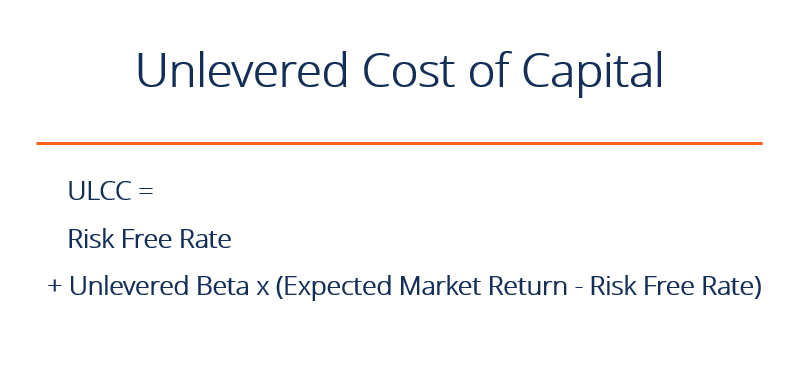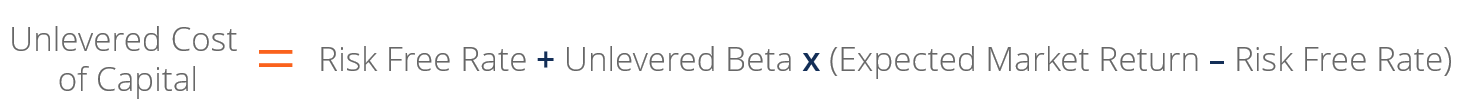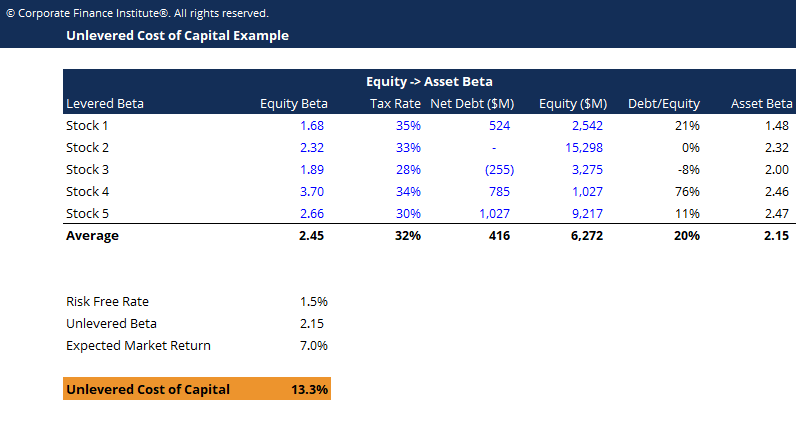# Unlevered Cost of Capital

The theoretical cost of a company financing itself, assuming no debt.

## What is the unlevered cost of capital?

The unlevered cost of capital is the implied rate of return a company expects to earn on its assets, without the effect of debt.

A company that wants to undertake a project will have to allocate capital or money for it. Theoretically, the capital could be generated either through debt or through equity. The weighted average cost of capital (WACC) assumes the company’s current capital structure is used for the analysis, while the unlevered cost of capital assumes the company is 100% equity financed.A hypothetical calculation is performed to determine the required rate of return on all-equity capital. This numerical figure or capital is the equity returns an investor expects the company to generate to justify the investment, given its risk profile. In reality, however, this number is just an assumption. Real figures cannot be given.

The theoretical cost is calculated using a formula. This gives an approximate of the likely requirement of the market.

### Calculating Unlevered Cost of Capital

To calculate a company’s unlevered cost of capital the following information is required:

• Risk-free Rate of Return
• Unlevered beta

The market risk premium is calculated by subtracting the expected market return and the risk free rate of return. Calculation of the firm’s risk premium is done by multiplying the company’s unlevered beta with the market risk premium.

Beta is the volatility of the stock versus the market, and the volatility of a stock is impacted by the amount of leverage the company has. The unlevered beta removes the effects of leverage from the company’s beta. Learn more.

### FormulaThis points to the theory that a company will have a higher unlevered cost of capital if investors perceive the stock as being higher risk.

### Unlevered cost of capital when there is debt

Ignoring the debt component and its cost is essential to calculate the company’s unlevered cost of capital, even though the company may actually have debt.

Now if the unlevered cost of capital is found to be 10% and a company has debt at a cost of just 5% then its actual cost of capital (WACC) will be lower than the 10% unlevered cost. This unlevered cost is still informative, but if the company fails to achieve the 10% unlevered returns that investors in this market require, then investor capital may move to alternative investments. This will lead to a fall in the company’s stock price.

### Unlevered Cost of Capital TemplateAs you can see in the example above, in order to calculate the unlevered cost of capital you need to determine the asset beta (the unlevered beta). Learn how to calculate it in CFI’s Guide to Calculating Unlevered Beta.

CFI is a global provider of the Financial Modeling & Valuation Analyst (FMVA)™ certification program and several other courses for finance professionals. To help you advance your career, check out the additional resources below:

• Cost of Preferred Stock
• Debt Schedule
• Weighted Average Cost of Capital
• Financial modeling guide

### Valuation Techniques

Learn the most important valuation techniques in CFI’s Business Valuation course!

Step by step instruction on how the professionals on Wall Street value a company.

Learn valuation the easy way with templates and step by step instruction!